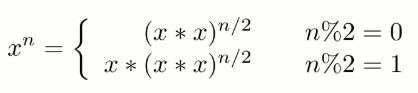# 16. 数值的整数次方

NowCoder

## 解题思路public double Power(double base, int exponent) {
if (exponent == 0)
return 1;
if (exponent == 1)
return base;
boolean isNegative = false;
if (exponent < 0) {
exponent = -exponent;
isNegative = true;
}
double pow = Power(base * base, exponent / 2);
if (exponent % 2 != 0)
pow = pow * base;
return isNegative ? 1 / pow : pow;
}